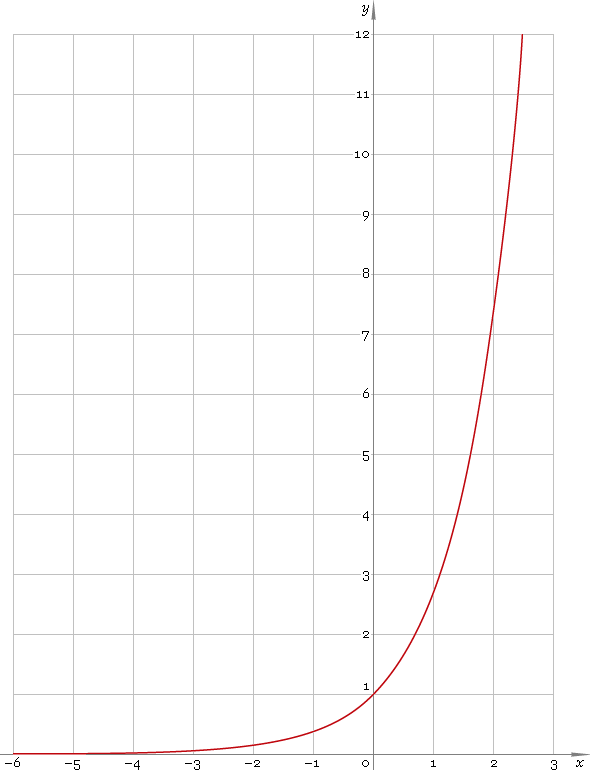The Art of Interface

# exp — exponential function

Category. Mathematics.

Abstract. Exponent: definition, plot, properties and identities.

## 1. Definition

Exponential function is the function of kind

ex

where constant e is selected so that slope of the function at point x = 0 is 45°.

## 2. Plot

Exponential function is defined everywhere on real axis. Its plot is depicted below — fig. 1.Fig. 1. Plot of the exponential function y = ex.

Function codomain is positive half of the real axis: (0, +∞).

## 3. Identities

By definition:

elnxx

Negative argument:

ex = 1 /ex

Sum and difference of arguments:

ex + y = ex ey
exy = ex/ey

Product of arguments:

exy = (ex)y

Base change:

ax = ex lna

## 4. Support

Exponential function exp of the real argument is supported by free version of the Librow calculator.

Exponential function exp of the complex argument is supported by professional version of the Librow calculator.

## 5. How to use

To calculate exponent of the number:

``exp(-1);``

To calculate exponent of the current result:

``exp(rslt);``

To calculate exponent of the number x in memory:

``exp(mem[x]);``